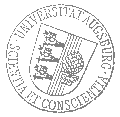# Universität AugsburgInstitut für MathematikOberseminar Numerische Mathematik

Dr. Théophile Chaumont-Frelet
Inria, Nizza

spricht am

Mittwoch, 19. Februar 2020

um

16:00 Uhr

im

Raum 1007 (L1)

über das Thema:

## »A multiscale hybrid-mixed method for the Helmholtz equation«

 Abstract: The Helmholtz equation models the propagation of a time-harmonic wave, which is a physical phenomenon at stake in several important applications, including radar, medical imaging, or resource prospecting. While this model is arguably simple, it is still challenging to numerically solve. Specifically, finite element schemes typically face two difficulties. On the one hand, the mesh size must satisfy drastic conditions when the wavelength $\lambda$ is small compared to the size of the domain $L$. On the other hand, the mesh size is also restricted by the characteristic length $\varepsilon$ of the heterogeneities of the medium, which is especially problematic in highly heterogeneous media. In this talk, we present a multiscale hybrid-mixed discretization scheme, which aims at overcoming these two challenges. Multiscale hybrid-mixed (or MHM, for short) methods are a family of finite element schemes for elliptic boundary value problems (BVP). These methods hinge on a hybrid variational formulation of the BVP. Given a partition of the domain, the solution $u$ is sought in a broken space $V$, while the continuity is weakly enforced through the action of a Lagrange multiplier $\lambda$. The Lagrange multiplier $\lambda$ lives in a space $\Lambda$ that is naturally associated with the faces of the partition. The fundamental observation behind the MHM formalism is that because there is no compatibility condition in the broken space $V$, the associated computations are local to each subdomain of the partition. This motivates the introduction of a multiscale Galerkin discrezation, which relies on finite-dimensional subspaces $V_h \subset V$ and $\Lambda_H \subset \Lambda$, where ideally $\varepsilon \simeq h \ll H \simeq \lambda \ll L$. In the first part of this talk, we present in detail the general ideas behind MHM methods. In a second part, we focus on the specificities associated with the Helmholtz equation. In particular, we carefully study the robustness of the method with respect to the number of wavelength in the domain $\lambda/L$. We finally present numerical experiments that highlight the key assets of the method, in particular in the case of highly heterogeneous media.

 Hierzu ergeht herzliche Einladung. Prof. Dr. Daniel Peterseim

[Impressum]      [Datenschutz]      wwwadm@math.uni-augsburg.de,     Fr 14-Feb-2020 09:59:49 MEZ